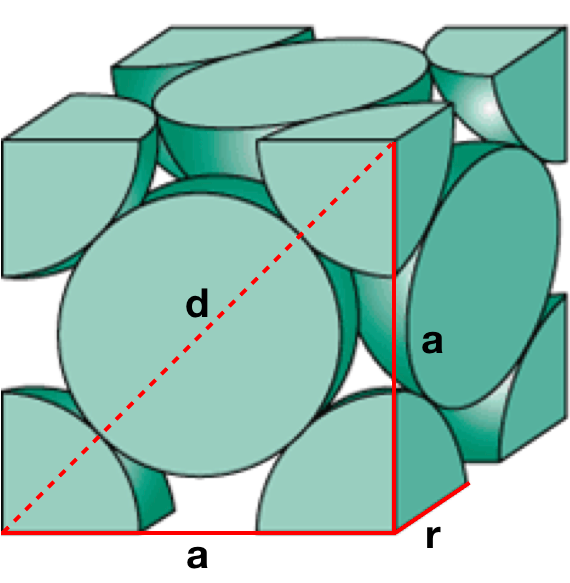# Problem: The density of solid Cu is 8.96 g/cm3. How many atoms are present per cubic centimeter of Cu? If a solid, Cu adopts a face-centered cubic unit cell. How many unit cells are present per cubic centimeter of Cu? What is the volume of a unit cell of this metal? What is the edge length of a unit cell of Cu?

###### FREE Expert Solution

Face-centered cubic lattice contains an atom in each of the face of the cube and an atom in each of the corners:a) Cu atoms per cm3

= 8.49 x 1022 Cu atoms/cm3

There are 8.49 x 1022 Cu atoms per cubic centimeter of Cu

b) Unit cells per 1 cmCu

Cu atoms per unit cell:

• corner atoms = 1/8  → 8 corners
• face atoms = 1/2 → 6 faces

4 Cu atoms/ unit cell

87% (114 ratings)###### Problem Details

The density of solid Cu is 8.96 g/cm3. How many atoms are present per cubic centimeter of Cu?

If a solid, Cu adopts a face-centered cubic unit cell. How many unit cells are present per cubic centimeter of Cu?

What is the volume of a unit cell of this metal?

What is the edge length of a unit cell of Cu?